## 0x00 Preface

Filesystem plays a critical role in one operating system. In this paper, I will go into details about the filesystem in Unix v6-plus-plus, which comes from Unix v6 and was modified in C-plus-plus to run on Bochs by our OS teachers for teaching.

We will call Unix v6-plus-plus as Unix v6pp.

### Content

I will descript the filesystem in 4 parts:

• Structure of files stored on the disc
• Structure of files opened in the memory
• Structure of file directory
• File operating interfaces

### Source Code Files

Files below are related: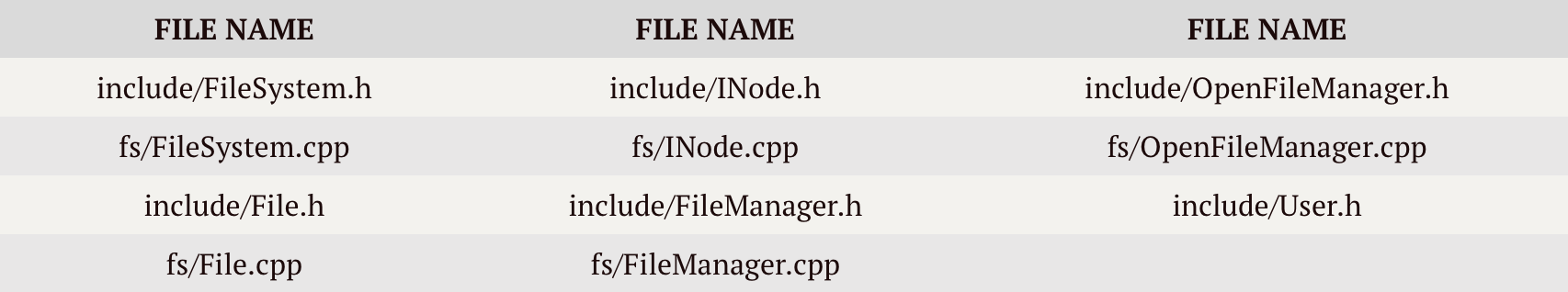Now, let’s be a Pirate :)

## 0x01 Structure of files stored on the disc

In this part, our goal is to explain how the files are organized on the disc. Files below are related:### Macro-Architecture

Let’s see some constants in the definition of `FileSystem` class:

``````// include/FileSystem.h
class FileSystem
{
public:
/* static consts */
static const int SUPER_BLOCK_SECTOR_NUMBER = 200;
static const int INODE_NUMBER_PER_SECTOR = 8;
static const int INODE_ZONE_START_SECTOR = 202;
static const int INODE_ZONE_SIZE = 1024 - 202;
static const int DATA_ZONE_START_SECTOR = 1024;
static const int DATA_ZONE_END_SECTOR = 18000 - 1;
static const int DATA_ZONE_SIZE = 18000 -  DATA_ZONE_START_SECTOR;
...
}
``````

From codes above we can draw up the macro-architecture with some extra knowledge about OS:

(unit: section)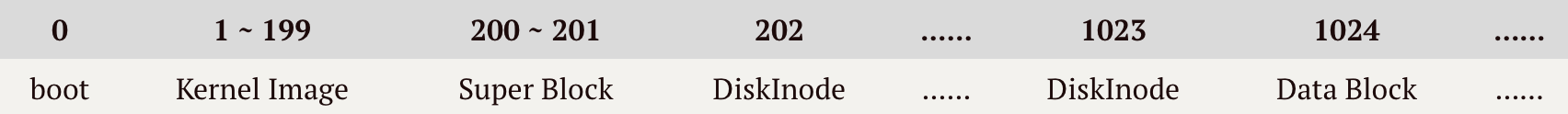Each section holds 512 bytes; each `DiskInode` is 64 bytes. So each section is able to hold 8 `DiskInode`s. `SuperBlock` is on 200 and 201 sections. `DiskInode`s occupy sections from 202 to 1023. Data blocks begin from NO.1024 section.

Unix v6pp does not manage sections from 0 to 199.

### SuperBlock

There is only one global `SuperBlock` object in the whole system. However, each block device has its own `SuperBlock`.

``````// fs/FileSystem.cpp
SuperBlock g_spb; // global object
``````

`SuperBlock` records information about a whole device. Let’s see the definition of `SuperBlock`:

``````// include/FileSystem.h
class SuperBlock
{
public:
SuperBlock(); // nothing to do
~SuperBlock(); // nothing to do
public:
int		s_isize;
int		s_fsize;
int		s_nfree;
int		s_free;
int		s_ninode;
int		s_inode;
int		s_flock;
int		s_ilock;
int		s_fmod;
int		s_ronly;
int		s_time;
};
``````

In Unix v6pp, Constructor function and Destructor function are both empty.

Now let’s talk about variables in this class.

First, these variables are easy to learn:

• `padding` enables `SuperBlock` to occupy 2 * 512 bytes (2 sections)
• `s_iszie` stores the number of sections used for `DiskInode`s
• `s_fsize` stores the number of sections
• `s_fmod` is a flag. If it is enabled, the `SuperBlock` on the disk should be update to that in the memory (modified)
• `s_ronly` is a flag, which means the filesystem is read-only
• `s_time` stores the time of the last updating operation

Second, the remaining variables are related to the management of free section blocks and free `DiskInode`s.

• `s_ninode` stores the number of free `DiskInode`s directly managed by `SuperBlock`
• `s_inode` is the index table of free `DiskInode`s directly managed by `SuperBlock`. The element in this array is the index of one `DiskInode` on one device
• `s_nfree` stores the number of free data blocks directly managed by `SuperBlock`. The element in this array is the index of one section block on one device
• `s_free`
• `s_ilock` is a lock to ensure mutex of different operations on `s_inode`
• `s_flock` is a lock to ensure mutex of different operations on `s_free`

The management of free `DiskInode`s and free section blocks is interesting. For convenience, `SuperBlock` does not directly manage all the free `DiskInode`s and free data blocks.

### Management of Free DiskInodes

`FileSystem::IAlloc(short dev)` and `FileSystem::IFree(short dev, int number)` are used to manage free `DiskInode`s:

#### Allocate

Firstly, `sb = this->GetFS(dev);` to fetch the `SuperBlock` of current device and make sure `s_ilock` is unlocked (or wait for it is unlocked):

``````sb = this->GetFS(dev);
while(sb->s_ilock){
u.u_procp->Sleep((unsigned long)&sb->s_ilock, ProcessManager::PINOD);
}
``````

If `s_inode` is empty, then lock `s_ilock` and search for free `DiskInode`s and record them into `s_inode[]` until `s_ninode` is 100 or no free `DiskInode` remains. If `IALLOC` of one `DiskInode` is disabled, system will then check whether that has been loaded into memory. Only these two conditions are met can one `DiskInode` prove to be free and be added into `s_inode[]`:

``````if(sb->s_ninode <= 0){
sb->s_ilock++;
ino = -1;
for(int i = 0; i < sb->s_isize; i++){
pBuf = this->m_BufferManager->Bread(dev, FileSystem::INODE_ZONE_START_SECTOR + i);
for(int j = 0; j < FileSystem::INODE_NUMBER_PER_SECTOR; j++){
ino++;
int mode = *( p + j * sizeof(DiskInode)/sizeof(int) );
if(mode != 0){
continue;
}
if( g_InodeTable.IsLoaded(dev, ino) == -1 ){
sb->s_inode[sb->s_ninode++] = ino;
if(sb->s_ninode >= 100)
{
break;
}
}
}
this->m_BufferManager->Brelse(pBuf);
if(sb->s_ninode >= 100){
break;
}
}
sb->s_ilock = 0;
Kernel::Instance().GetProcessManager().WakeUpAll((unsigned long)&sb->s_ilock);
if(sb->s_ninode <= 0){
Diagnose::Write("No Space On %d !\n", dev);
u.u_error = User::ENOSPC;
return NULL;
}
}
``````

If system reaches here, there must remain free `DiskInode` in `s_inode[]`. Load all the `DiskInode`s in `s_inode[]` into memory until `INodeTable` is full:

``````while(true){
ino = sb->s_inode[--sb->s_ninode];
pNode = g_InodeTable.IGet(dev, ino);
if(NULL == pNode){
return NULL;
}
if(0 == pNode->i_mode){
pNode->Clean();
sb->s_fmod = 1;
return pNode;
}
else{
g_InodeTable.IPut(pNode);
continue;
}
}
``````

Attention! When system modifies `SuperBlock` in the memory, it should enable `s_fmod`.

#### Free

This function is easy to understand. When `s_ilock` is unlocked and `s_ninode` is less than 100, then record `DiskInode` indexed by `number` in `s_inode`, or just return.

``````void FileSystem::IFree(short dev, int number)
{
SuperBlock* sb;
sb = this->GetFS(dev);
if(sb->s_ilock){
return;
}
if(sb->s_ninode >= 100){
return;
}
sb->s_inode[sb->s_ninode++] = number; // push
sb->s_fmod = 1;
}
``````

### Management of Free Data Blocks

Unix v6pp uses grouping chained index table to manage free data blocks. Picture below simply shows the structure: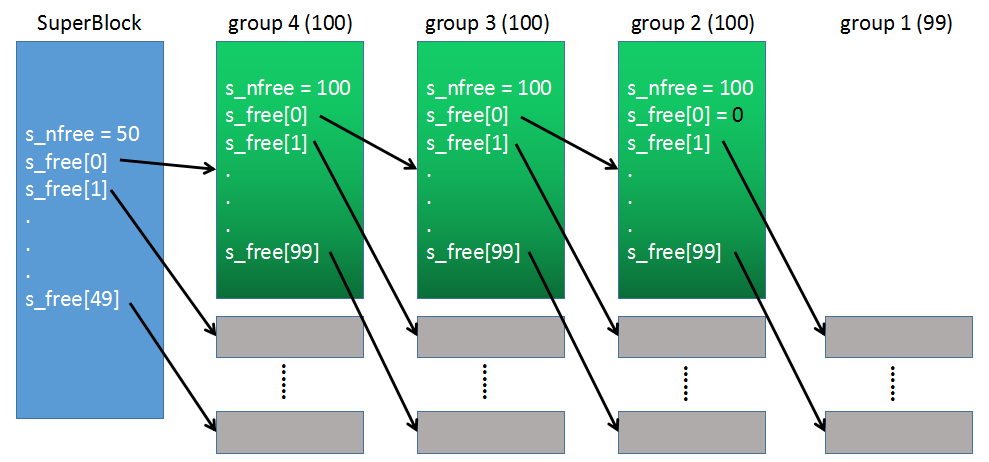`FileSystem::Alloc(short dev)` and `FileSystem::Free(short dev, int blkno)` are used to manage free data blocks:

#### Allocate

When `s_flock` is unlocked, use `blkno` to fetch one free data block. If `blkno` is 0, there is no more free data block, then return. If `blkno` is invalid (checked by `BadBlock()`), then return.

``````sb = this->GetFS(dev);
while(sb->s_flock){
u.u_procp->Sleep((unsigned long)&sb->s_flock, ProcessManager::PINOD);
}
blkno = sb->s_free[--sb->s_nfree];
if(0 == blkno ){
sb->s_nfree = 0;
Diagnose::Write("No Space On %d !\n", dev);
u.u_error = User::ENOSPC;
return NULL;
}
return NULL;
}
``````

After `blkno` fetches one, if `s_nfree` is 0, then copy first 404 bytes (`s_nfree` + `s_free`) from data block with the index of `SuperBlock->s_free` to `SuperBlock->s_nfree` and `SuperBlock->s_free`, that is, take down the indirect index table of the next group (e.g. group 4 in the picture above):

``````if(sb->s_nfree <= 0){
sb->s_flock++;
sb->s_nfree = *p++;
Utility::DWordCopy(p, sb->s_free, 100);
this->m_BufferManager->Brelse(pBuf);
sb->s_flock = 0;
Kernel::Instance().GetProcessManager().WakeUpAll((unsigned long)&sb->s_flock);
}
pBuf = this->m_BufferManager->GetBlk(dev, blkno);
this->m_BufferManager->ClrBuf(pBuf);
sb->s_fmod = 1;

return pBuf;
``````

#### Free

Firstly wait until `s_flock` is unlocked and blkno is valid:

``````sb = this->GetFS(dev);
sb->s_fmod = 1;
while(sb->s_flock){
u.u_procp->Sleep((unsigned long)&sb->s_flock, ProcessManager::PINOD);
}
return;
}
``````

If `blkno` is going to be the first free data block, use `s_free` to mark end and use `s_free` to point to this `blkno` data block; else, copy `SuperBlock->s_nfree` and `SuperBlock->s_free` (404 bytes) to `blkno` data block, then use `SuperBlock->s_free` to point to `blkno` data block and set `SuperBlock->s_nfree` to 1:

``````if(sb->s_nfree <= 0){
sb->s_nfree = 1;
sb->s_free = 0;
}
if(sb->s_nfree >= 100){
sb->s_flock++;
pBuf = this->m_BufferManager->GetBlk(dev, blkno);
*p++ = sb->s_nfree;
Utility::DWordCopy(sb->s_free, p, 100);
sb->s_nfree = 0;
this->m_BufferManager->Bwrite(pBuf);
sb->s_flock = 0;
Kernel::Instance().GetProcessManager().WakeUpAll((unsigned long)&sb->s_flock);
}
sb->s_free[sb->s_nfree++] = blkno;
sb->s_fmod = 1;
``````

### File Structure

In Unix, everything is a file. So In this sub-part we just talk about the conception of File on the layer of `DiskInode-DataBlock` model, without the specific file meanings and structures. In this model, one file is organised as meta-data and file-data: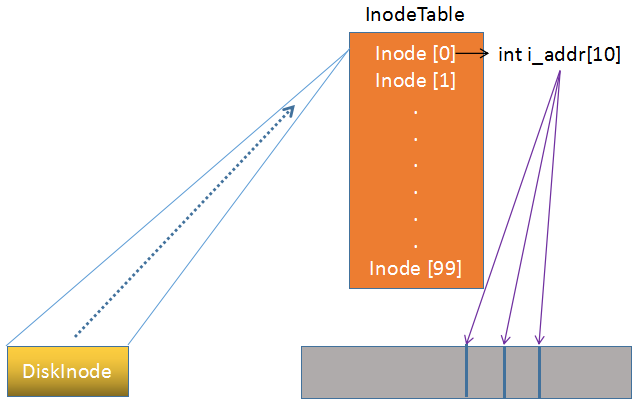Meta data helps to manage the data blocks. So let’s see the definition of `DiskInode` class:

``````// include/INode.h
class DiskInode
{
public:
DiskInode();
~DiskInode(); // nothing to do
public:
unsigned int d_mode;
short	d_uid;
short	d_gid;
int		d_size;
int		d_atime;
int		d_mtime;
};
``````
``````// fs/Inode.cpp
DiskInode::DiskInode()
{
this->d_mode = 0;
this->d_uid = -1;
this->d_gid = -1;
this->d_size = 0;
for(int i = 0; i < 10; i++){
}
this->d_atime = 0;
this->d_mtime = 0;
}
``````

`DiskInode::DiskInode()` is to initialize variables in the class. This is Necessary. When one `DiskInode` is in the stack, not all entries will be updated. So when `sync` is operated you should set variables not updated to default values instead of values remaining on the stack before this `DiskInode` is loaded.

• `d_mode` records states of one file, lower 16 bits used: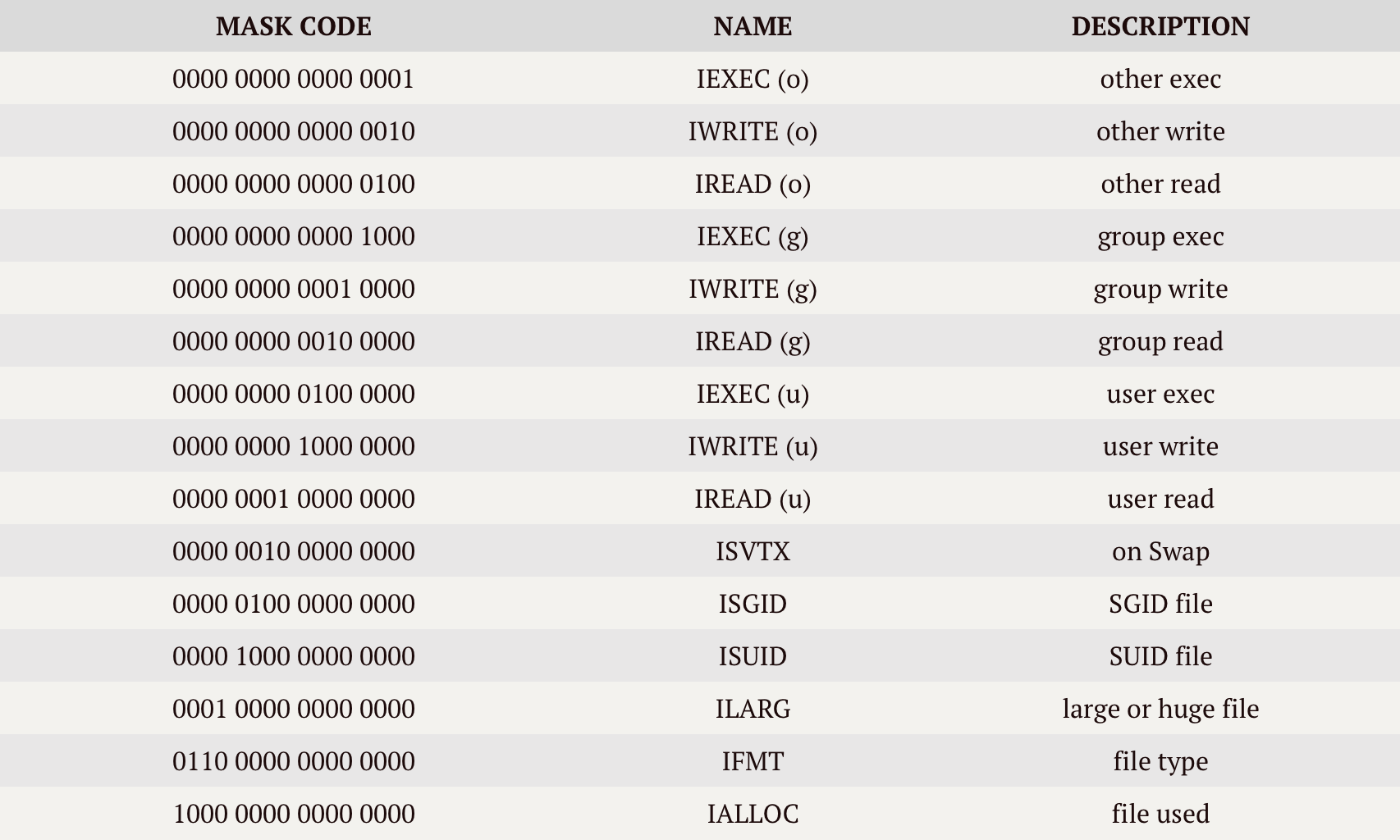Definitions above can be found in `INode` class, We will talk about which in 0x02 part.

More about `IFMT`:

``````00 - Common data file
01 - Character device file
10 - Directory file
11 - Block device file
``````
• `d_nlink` counts the number of different path names for one file in the whole directory tree (That is, hard link)
• `d_uid` stores the owner’s ID
• `d_gid` stores the owner group’s ID
• `d_atime` stores the last access time
• `d_mtime` stores the last modified time
• `d_size` stores the size of one file (unit: byte)

From `INode` class we find some constants:

``````static const int BLOCK_SIZE = 512;
static const int ADDRESS_PER_INDEX_BLOCK = BLOCK_SIZE / sizeof(int);
static const int SMALL_FILE_BLOCK = 6;
static const int LARGE_FILE_BLOCK = 128 * 2 + 6;
static const int HUGE_FILE_BLOCK = 128 * 128 * 2 + 128 * 2 + 6;
``````

Now we can calculate and sum these data:(unit: byte)

Now we can talk about `d_addr`. This array stores index of data blocks related.

If one is a small file, this array will be: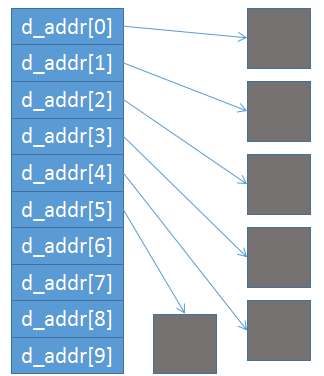If one is a large file, this array will be: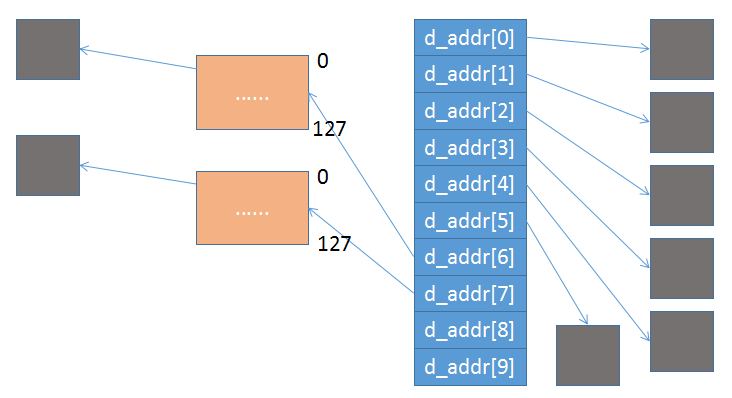Note that 1 level indirect index blocks appear.

If one is a huge file, this array will be: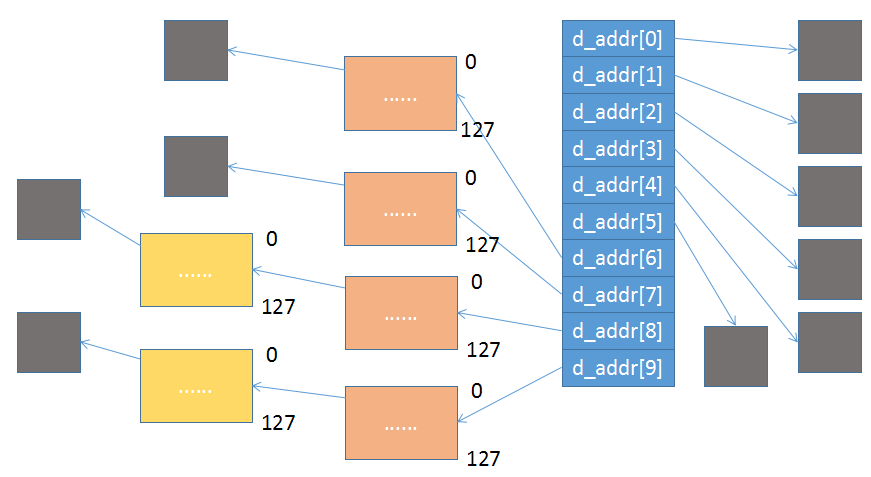Note that 2 level indirect index blocks appear.

`d_size` determines whether one is a small, large or huge file.

## 0x02 Structure of files opened in the memory

In this part, our goal is to descript structures of files opened in the memory. Files below are related: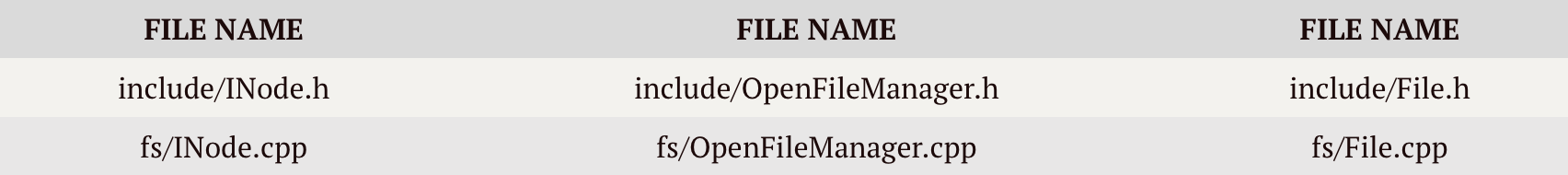Exactly, we also need another file `include/User.h`, but actually we only need two statements in `User` class:

``````OpenFiles u_ofiles;
IOParameter u_IOParam;
``````

We only need to know that `OpenFiles` and `IOParameter` are in this class, because `User` class is the extended control block of one process.

We have learnt how files are stored on the disk. That is very very good.

### Integral Comprehension

Firstly, we’d better have an integral comprehension: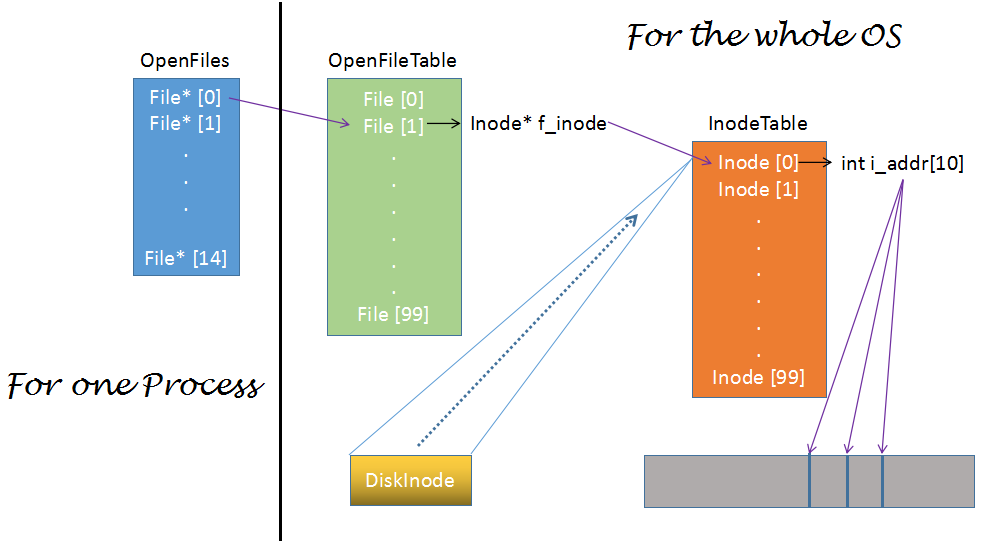You may note that all these structures have nothing to do with filename. That is because the filename is stored in directory files.

The index of entries in `OpenFiles` is the famous `fd` (file descriptor).

It will be comprehensible if we talk about this topic step by step because we can show a dynamic process.

### From DiskInode to INodeThe picture above means that we will map one `DiskInode` on the disk to one `Inode` in the memory.

First, let’s study the `Inode` class, whose variables are very similar to those in `DiskInode` class: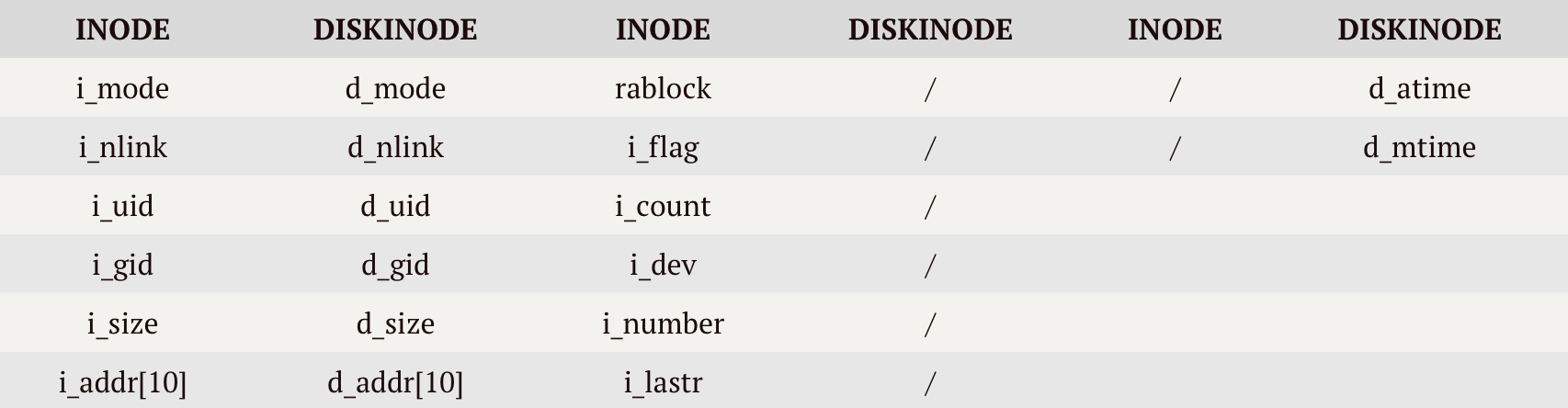The duplicate variables won’t be explained. We focus on the new in `Inode`:

• `i_dev` stores ID of the device from which one `DiskInode` comes
• `i_number` stores ID of one `DiskInode` on a disk
• `i_flag` stores some flags: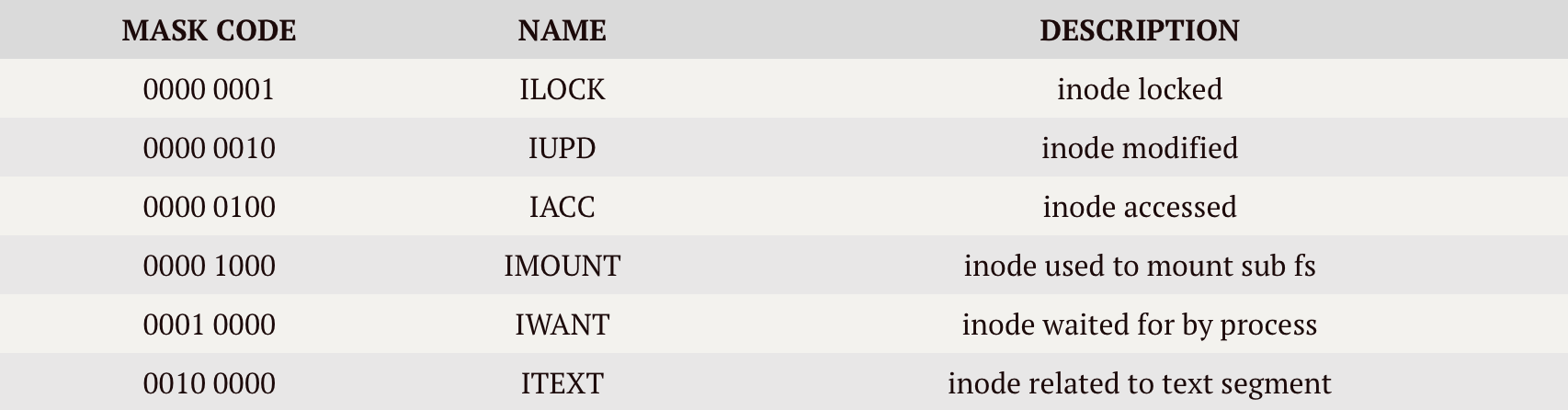• `i_count` stores number of instances referencing this inode. If it is 0, this inode is free
• `i_lastr` stores logic ID of the last block read to judge whether to do read-ahead operation
• `rablock` stores physical ID of the next block for read-ahead

`Inode` does not care about `d_atime` and `d_mtime`.

Attention! Relationship between one `Inode` and one `DiskInode` is one-to-one.

`Inode` class provides some important methods:

``````int Bmap(int lbn);
void WriteI();
``````

Bmap

`Bmap` is to translate logic block number (lbn) into physical block number (phyBlkno), on which other methods rely. Exactly, `lbn` is the index of one entry in `i_addr ~ i_addr` for small files, `i_addr ~ i_addr + extra 1 level indirect index block` for large files or `i_addr ~ i_addr + extra 1 level indirect index block + 2 level indirect index block` for huge files. `phyBlkno` is the value of such an entry.

The structure of `Bmap` is very clear (in pseudocode):

``````if lbn >= HUGE_FILE_BLOCK, return (lbn invalid)
if lbn < 6 (small file)
if phyBlkno is 0 then allocate
if allocate successfully
phyBlkno = pFirstBuf->b_blkno (fetch number)
else (fail to allocate)
return phyBlkno
else (large/huge file)
use lbn to calculate index1 in i_addr[]
if phyBlkno is 0 then allocate 1 level indirect index block
if failed then return
read the 1 level indirect index block and point by iTable
if this is a huge file
use lbn to calculate index2 in 1 level indirect index block
phyBlkno = iTable[index2]
if phyBlkno is 0 then allocate 2 level block
read the 2 level block and point by iTable
phyBlkno = iTable[index] (if 0 then allocate)
return phyBlkno
``````

This method is to read file data.

``````if u_IOParam.m_Count is 0 then return (nothing rest)
if inode is char device then invoke Read() of CharDevice and return
while (no error and u_IOParam.m_Count is not 0)
fetch dev and use Bmap to get bn
IOMove(start, u.u_IOParam.m_Base, nbytes) (copy to user)
update u_IOParam
``````

WriteI

This method is to write data to file.

``````if inode is char device then invoke Write() of CharDevice and return
if u_IOParam.m_Count is 0 then return (nothing rest)
while (no error and u_IOParam.m_Count is not 0)
fetch dev and use Bmap to get bn
if data to write is 512 bytes, then allocate buffer
calculate where to write: start = pBuf->b_addr + offset
IOMove(u.u_IOParam.m_Base, start, nbytes) (copy)
update u_IOParam variables
if one data block is full then rsync write (Bawrite)
else delay and write (Bdwrite)
update i_size (file's size)
``````

Now you have learnt most of `Inode` class. Let’s continue to see how one `DiskInode` is mapped to one `Inode`.

This process is mainly operated by `Inode* IGet(short dev, int inumber)` in `InodeTable`. In Unix v6pp, `InodeTable` has an inode array `m_Inode[NINODE]` (`NINODE` = 100). `IGet()` is to map `DiskInode` to one `Inode`. In addition, `IPut()` is to decrease `i_count` or free one `Inode`.

`Inode* InodeTable::IGet(short dev, int inumber)`:

``````while
pInode = &(this->m_Inode[index]) (try to fetch it)
if inode locked, then want it and sleep
if sub-fs is mounted at this inode, then
dev = pMount->m_dev (fetch real device number)
inumber = FileSystem::ROOTINO (fetch real inode number)
continue in while
pInode->i_count++ (reference number increase)
lock
return pInode
else
GetFreeInode()
if allocate successfully
set i_dev/i_number/i_flag/i_count/i_lastr
if I/O error occurs, release buffer and IPut()
pInode->ICopy(pBuf, inumber) (copy to Inode)
release buffer
return pInode
``````

We can have short look at `Inode::ICopy()`:

``````void Inode::ICopy(Buf *bp, int inumber)
{
DiskInode dInode;
DiskInode* pNode = &dInode;
unsigned char* p = bp->b_addr + (inumber % FileSystem::INODE_NUMBER_PER_SECTOR) * sizeof(DiskInode);
Utility::DWordCopy( (int *)p, (int *)pNode, sizeof(DiskInode)/sizeof(int) );
this->i_mode = dInode.d_mode;
this->i_uid = dInode.d_uid;
this->i_gid = dInode.d_gid;
this->i_size = dInode.d_size;
for(int i = 0; i < 10; i++){
}
}
``````

Very easy, right?

By the way, I want to show you the process of `IPut()`. When one file is `close()`, `IPut` will be invoked.

``````if pNode->i_count == 1 (only one reference)
lock
if i_nlink <=0 (no directory path points to it)
ITrunc() (truncate data block)
i_mode = 0
IFree(pNode->i_dev, pNode->i_number) (free DiskInode)
IUpdate(Time::time) (update DiskInode)
Prele() (unlock Inode)
i_flag = 0
i_number = -1
i_count--
Prele() (unlock Inode)
``````

So far, we have mapped `DiskInode` to `Inode`.

### Between OpenFileTable and InodeTable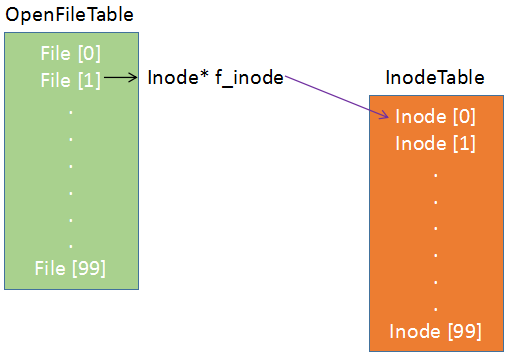Here we begin with the system call `SystemCall::Sys_Open()`. This API is famous and clear, and we will dive into something else interesting :)

In `SystemCall::Sys_Open()`:

``````fileMgr.Open();
``````

`fileMgr` is an object of `FileManager` class, which has three important pointers:

``````FileSystem* m_FileSystem;
InodeTable* m_InodeTable;
OpenFileTable* m_OpenFileTable;
``````

So you can see it is the very chief. Now let’s see `FileManager::Open()`:

``````this->Open1(pInode, u.u_arg, 0);
``````

As we see, It has Another `FileManager::Open1()` used not only by `FileManager::Open()` but also by `FileManager::Creat()`. Dive into it and we can see:

``````File* pFile = this->m_OpenFileTable->FAlloc();
if ( NULL == pFile )
{
this->m_InodeTable->IPut(pInode);
return;
}
pFile->f_flag = mode & (File::FREAD | File::FWRITE);
pFile->f_inode = pInode;
``````

Note that `pFile->f_inode = pInode` connects `Inode` with `File`.

### Between ProcessOpenFileTable and OpenFileTable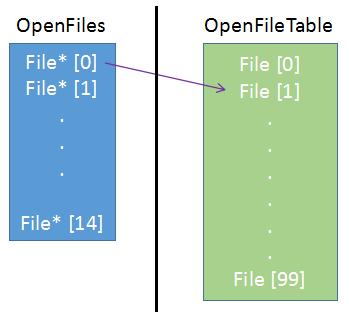Here we are interested in `OpenFileTable`. In Unix v6pp, `m_File[NFILE]` in it has 100 `File` objects.

`File* FAlloc()` is to allocate one free `File` in `m_File[]`.

``````fd = u.u_ofiles.AllocFreeSlot() (find one free File* in Process)
if fd < 0 return NULL (Process is unable to open more file)
for(int i = 0; i < OpenFileTable::NFILE; i++)
if(this->m_File[i].f_count == 0) (free to use)
u.u_ofiles.SetF(fd, &this->m_File[i])
this->m_File[i].f_count++
this->m_File[i].f_offset = 0
return (&this->m_File[i])
return NULL
``````

Note that `u.u_ofiles.SetF(fd, &this->m_File[i])` connects `File*` in `OpenFiles` with `File` in `OpenFileTable`.

We can dive into `OpenFiles::SetF(int fd, File* pFile)`:

``````	if(fd < 0 || fd >= OpenFiles::NOFILES){
return;
this->ProcessOpenFileTable[fd] = pFile;
``````

All the relationship in the integral picture has been talked about. At last, we will explore `OpenFileTable::CloseF(File *pFile)`, which is to decrease `f_count` or free one `File`:

``````if(pFile->f_flag & File::FPIPE){
pNode = pFile->f_inode;
procMgr.WakeUpAll((unsigned long)(pNode + 1));
procMgr.WakeUpAll((unsigned long)(pNode + 2));
}
``````

Code above is to deal with `pipe` which we Currently do not analyse.

``````if(pFile->f_count <= 1)
pFile->f_inode->CloseI(pFile->f_flag & File::FWRITE);
g_InodeTable.IPut(pFile->f_inode);
}
``````

if `f_count` <= 1 then current process is the last process referencing this `File`. For special block device or char device invoke `CloseI`. For common file, just invoke `IPut` which we have talked about before.

Finally,

``````pFile->f_count--;
``````

In `File` there is a `f_count` and in `Inode` there is a `i_count`. This idea is graceful.

## 0x03 Structure of file directory

In this part, our goal is to descript structures of directory file.

Files below are related: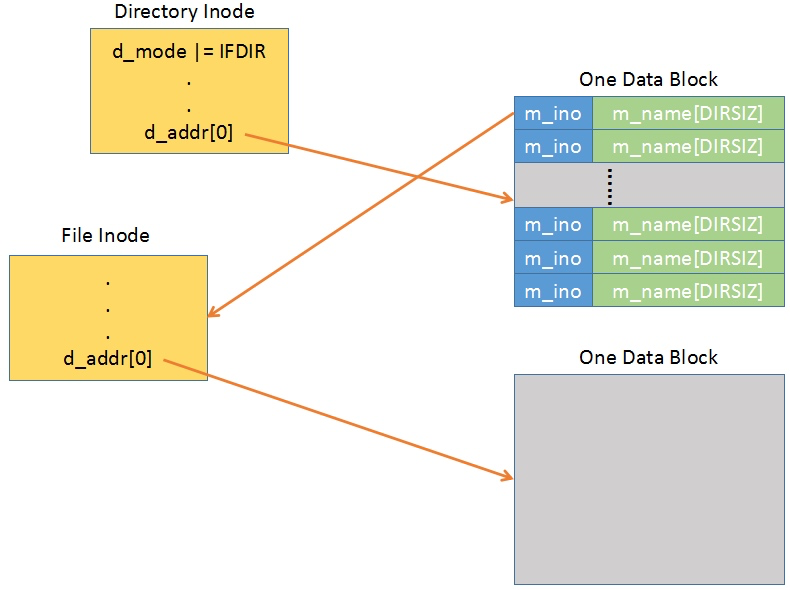To some extent, the picture above is enough to explain this part. But for completeness, some other explanations are added:

Everything is a file, so is the directory. The data block of one directory file stores `inode-filename` entries. And follow this clue, you can find the inode of one specific file with a specific name.

That’s all, thank you :)

## 0x04 File operating interfaces

In this part, our goal is to descript File Operating Interface Files below are related:I plan to analyse these methods:

``````void FileManager::Open()
void FileManager::Creat()
void FileManager::Open1(Inode* pInode, int mode, int trf)
void FileManager::Close()
void FileManager::Seek()
void FileManager::Write()
void FileManager::Rdwr(enum File::FileFlags mode)
Inode* FileManager::NameI(char (*func)(), enum DirectorySearchMode mode)
``````

There are also some other important methods in `FileManager`. But here we currently care about I/O related. And we follow the general operating order, that is, first open, then seek one position, then read or write and finally close one file.

### Open/Create/Open1/NameI

The relationship among these 4 methods is interesting! See the picture below: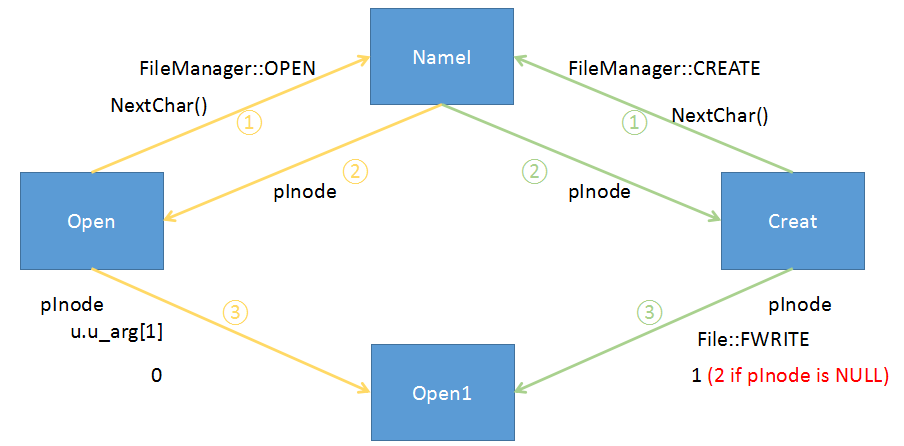`NextChar()` is a method to return the next `char` in pathname. If `NameI` return NULL, then `Open` will directly return without calling `Open1`, while `Creat` will call `MakNode` to return a new `Inode` to `pInode` if no error occurs. And `Creat` will do `pInode->i_mode |= newACCMode`.

NameI

Now dive into `NameI`. This method is so important that it translates pathname to `Inode`. But this method is complex, so be patient :)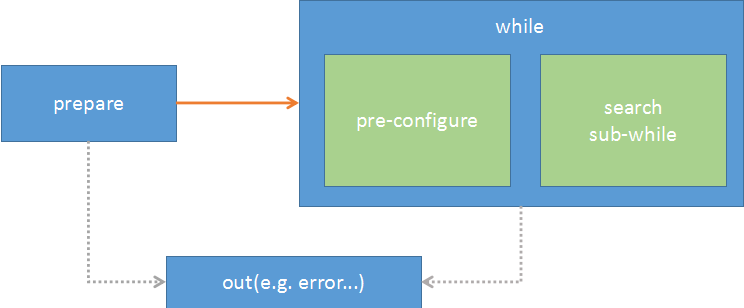The out part only has two statements:

``````this->m_InodeTable->IPut(pInode);
return NULL;
``````

When error occurs, `goto out` will be done.

In preapre part, it is very clear:

``````pInode = u.u_cdir;
if ( '/' == (curchar = (*func)()) )
pInode = this->rootDirInode;
this->m_InodeTable->IGet(pInode->i_dev, pInode->i_number);
while ( '/' == curchar )
curchar = (*func)();
if ( '\0' == curchar && mode != FileManager::OPEN ){
u.u_error = User::ENOENT;
goto out;
}
``````

With pathname like `/home/temp`, `pInode` will be assigned `rootDirInode`; with `home/temp`, `pInode` will be current directory. With `///home/temp`, `//` is skipped. And if you want to modify the current directory, error occurs and `goto out`.

We will take a pathname example: `/home/temp`.

Before program goes into `while`, `pInode` points to `Inode` of `/` and `curchar` is `h`.

In `while`, first do some `pre-configure`:

``````if (u.u_error != User::NOERROR)
break;	/* error, goto out; */
if ('\0' == curchar)
return pInode; // succeed, return
if ( (pInode->i_mode & Inode::IFMT) != Inode::IFDIR ){
u.u_error = User::ENOTDIR;
break;	/* not dir, goto out; */
}
if ( this->Access(pInode, Inode::IEXEC) ){
u.u_error = User::EACCES;
break; /* no search right, goto out; */
}
``````

Then copy `home` to `u.u_dbuf` and `curchar` now stores `t`.

Before search:

``````u.u_IOParam.m_Offset = 0;
u.u_IOParam.m_Count = pInode->i_size / (DirectoryEntry::DIRSIZ + 4);
freeEntryOffset = 0;
pBuf = NULL;
``````

Now search `home` in `/`’s directory entry in a `sub-while`:

``````while (true){
if ( 0 == u.u_IOParam.m_Count ){ // search over
if ( NULL != pBuf )
bufMgr.Brelse(pBuf);
// if create new file
if ( FileManager::CREATE == mode && curchar == '\0' ){
// check whether have right to write
if ( this->Access(pInode, Inode::IWRITE) ){
u.u_error = User::EACCES;
goto out;
}
// store parent inode for WriteDir()
u.u_pdir = pInode;
if ( freeEntryOffset )
u.u_IOParam.m_Offset = freeEntryOffset - (DirectoryEntry::DIRSIZ + 4); // store offset for WriteDir()
else
pInode->i_flag |= Inode::IUPD;
return NULL; // find the free entry so return
}
u.u_error = User::ENOENT;
goto out;
}
if ( 0 == u.u_IOParam.m_Offset % Inode::BLOCK_SIZE ){
if ( NULL != pBuf )
bufMgr.Brelse(pBuf);
int phyBlkno = pInode->Bmap(u.u_IOParam.m_Offset / Inode::BLOCK_SIZE );
}
// read the next directory entry into u.u_dent
int* src = (int *)(pBuf->b_addr + (u.u_IOParam.m_Offset % Inode::BLOCK_SIZE));
Utility::DWordCopy( src, (int *)&u.u_dent, sizeof(DirectoryEntry)/sizeof(int) );
u.u_IOParam.m_Offset += (DirectoryEntry::DIRSIZ + 4);
u.u_IOParam.m_Count--;
if ( 0 == u.u_dent.m_ino ){ // skip empty entry
if ( 0 == freeEntryOffset )
freeEntryOffset = u.u_IOParam.m_Offset;
continue;
}
int i;
// compare entry string
for ( i = 0; i < DirectoryEntry::DIRSIZ; i++ )
if ( u.u_dbuf[i] != u.u_dent.m_name[i] )
break;
if( i < DirectoryEntry::DIRSIZ ) // not the same
continue;
else
break; // same, break
}
``````

We should pay attention to some variables: `pInode` points to current directory we search in; `curchar` points to the next `char` in current part of path; `u.u_dbuf[]` stores string we look for; `u.u_dent.m_name[]` stores one directory entry’s name.

If the `sub-while` is `break`, it means part matches successfully. And go ahead:

``````// if this is DELETE operation
if ( FileManager::DELETE == mode && '\0' == curchar ){
if ( this->Access(pInode, Inode::IWRITE) ){
u.u_error = User::EACCES;
break;	/* goto out; */
}
return pInode;
}
``````

Arriving here, there is a `home` entry in `/`. So program will dive into `home` and continue:

``````short dev = pInode->i_dev;
this->m_InodeTable->IPut(pInode);
pInode = this->m_InodeTable->IGet(dev, u.u_dent.m_ino);
if ( NULL == pInode )
return NULL;
``````

`NameI` is complex, but not awesome.

Open1

Now let’s dive into `Open1` and see it from the view of methods and classes: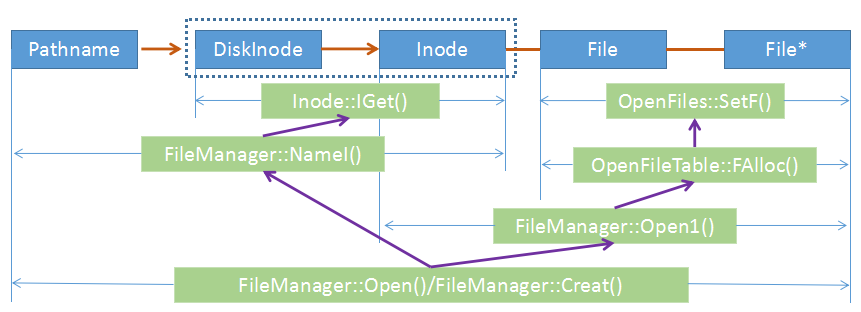### Seek

We all know the function of `Seek`. Now let’s see how it make it.

``````int fd = u.u_arg;
pFile = u.u_ofiles.GetF(fd);
if ( NULL == pFile ) // no such file in memory (maybe not open)
return;
``````

`PIPE` file is not allowed to be sought:

``````if ( pFile->f_flag & File::FPIPE ){
u.u_error = User::ESPIPE;
return;
}
``````

Unit of length will change from byte to 512 bytes if u_arg > 2:

``````int offset = u.u_arg;
if ( u.u_arg > 2 ){
offset = offset << 9;
u.u_arg -= 3;
}
``````

Code below sets the r/w offset:

``````switch ( u.u_arg ){
case 0:
pFile->f_offset = offset;
break;
case 1:
pFile->f_offset += offset;
break;
case 2:
pFile->f_offset = pFile->f_inode->i_size + offset;
break;
}
``````

`Read`:

``````this->Rdwr(File::FREAD);
``````

`Write`:

``````this->Rdwr(File::FWRITE);
``````

So let’s see `Rdwr`:

``````pFile = u.u_ofiles.GetF(u.u_arg); /* fd */
if ( NULL == pFile )
return;
if ( (pFile->f_flag & mode) == 0 ){ // r/w mode invalid
u.u_error = User::EACCES;
return;
}
u.u_IOParam.m_Base = (unsigned char *)u.u_arg;
u.u_IOParam.m_Count = u.u_arg; // r/w bytes
u.u_segflg = 0;
if(pFile->f_flag & File::FPIPE){ // pipe r/w
if ( File::FREAD == mode )
else
this->WriteP(pFile);
}
else{
pFile->f_inode->NFlock();
u.u_IOParam.m_Offset = pFile->f_offset; // set offset
if ( File::FREAD == mode )
else
pFile->f_inode->WriteI();
pFile->f_offset += (u.u_arg - u.u_IOParam.m_Count); // update offset
pFile->f_inode->NFrele();
}
u.u_ar0[User::EAX] = u.u_arg - u.u_IOParam.m_Count;
``````

### Close

``````void FileManager::Close()
{
User& u = Kernel::Instance().GetUser();
int fd = u.u_arg;

File* pFile = u.u_ofiles.GetF(fd);
if ( NULL == pFile )
return;
u.u_ofiles.SetF(fd, NULL);
this->m_OpenFileTable->CloseF(pFile);
}
``````

Use `pFile` to fetch the `File` structure and set `File*` in `OpenFiles` to `NULL` then call `CloseF`.